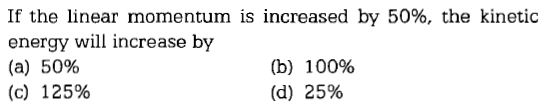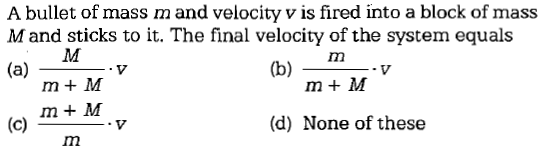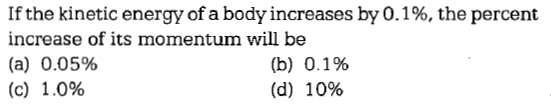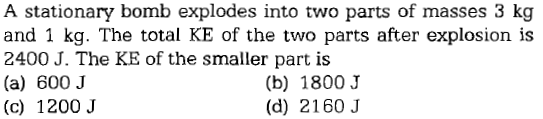#6 | Problems
(Physics) > Systems of Particles and Rotational Motion
Related Practice Questions :

A block of mass m moving at a velocity v collides with another block of mass 2m at rest. The lighter block comes to rest after collision. Find the coefficient of restitution.

(a) $\frac{1}{2}$                                               (b) $1$

(c) $\frac{1}{3}$                                               (d) $\frac{1}{4}$

Complete Question Bank + Test Series
Complete Question Bank

Difficulty Level:Complete Question Bank + Test Series
Complete Question Bank

Difficulty Level:Complete Question Bank + Test Series
Complete Question Bank

Difficulty Level: